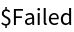Function Repository Resource:

# ColorWinding

Find the number of times the domain coloring of a function wraps around the color wheel along a given curve

Contributed by: Garrett Dubofsky, Wolfram|Alpha Math Team
 ResourceFunction["ColorWinding"][{f1,f2},{x,y},{p1,p2,…}] finds the winding number of the 2D transformation {f1,f2} with variables {x,y} along the path formed by linearly connecting the points pi. ResourceFunction["ColorWinding"][{f1,f2},{x,y},{{p11,p12,…},…}] finds the winding number over a collection of curves. ResourceFunction["ColorWinding"][{f1,f2},{x,y},points, output] specifies the output type using output. ResourceFunction["ColorWinding"][{f1,f2},{x,y},"DomainColor"] outputs the domain coloring of the transformation.

## Details

A domain coloring pairs every transformed point’s angle around the origin to a specific hue. This is a method used to visualize 2D transformations. The red hue lies along the negative y-axis and the color wheel is oriented counterclockwise around the origin.
The winding number of a 2D function along a curve is the number of times the domain coloring of the function winds around the color wheel as the curve is transversed.
Wrapping in the order of red green blue represents a positive winding number, whereas blue green red is negative.
The winding number is an integer.
Both functions f1 and f2 should depend on the independent variables x and y and output a single numeric value.
The value of output can be a single item or a list containing any of "Number", "HuePlot", "PolygonPlot", "DomainColor", or All.
If output is set to All, the function returns an Association.
ResourceFunction["ColorWinding"] takes all the options for ParametricPlot with the following additions:
 "ClosedCurve" True whether to connect the first and last points "IterationLength" 1/100 how frequently the Hue value is sampled "HueShift" 0 shift all colors around the Hue wheel

## Examples

### Basic Examples (5)

After the identity mapping is applied, the domain coloring of the unit square centered at the origin winds around the color wheel once:

 In:=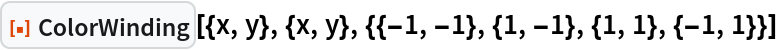Out=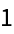The domain coloring along this curve can be visualized:

 In:=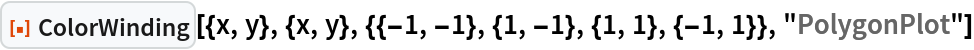Out=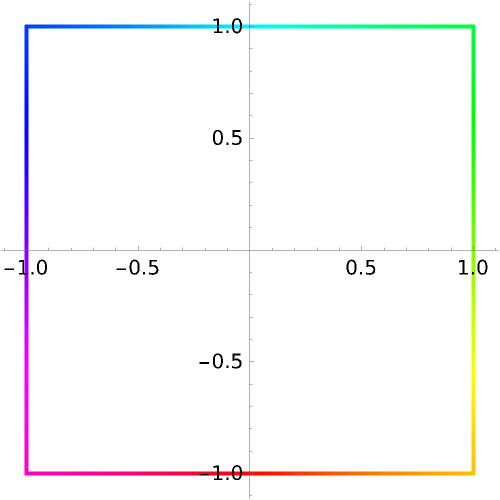The "HuePlot" output plots the domain coloring Hue value along the curve:

 In:=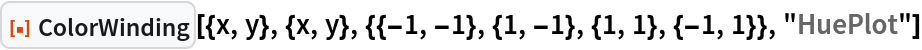Out=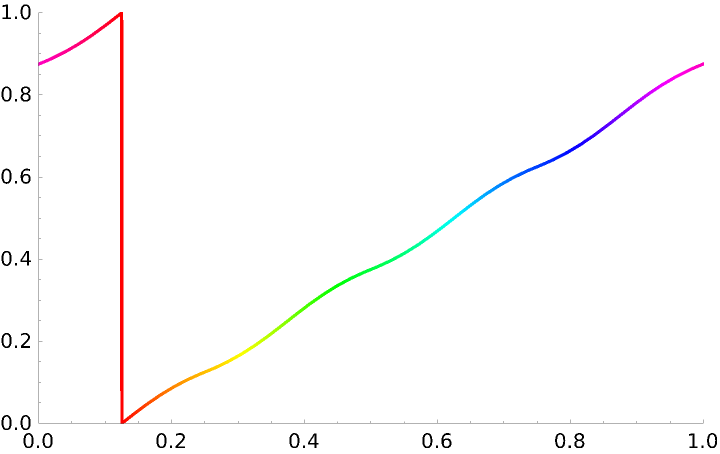Over the entire plane, the domain coloring of the identity mapping preserves the color wheel centered at the origin:

 In:=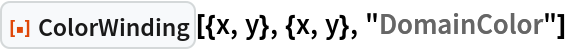Out=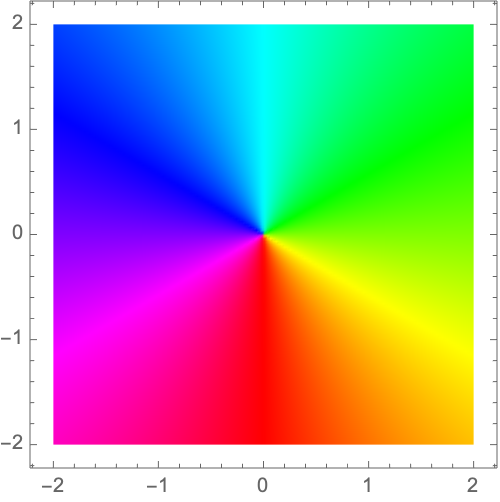If the order of the points is reversed, the winding number is negated:

 In:=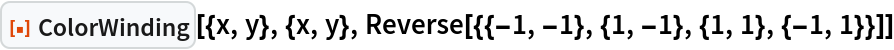Out=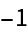### Scope (4)

Each 2D transformation produces its own domain coloring:

 In:=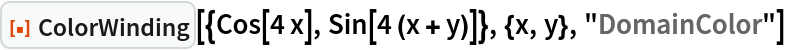Out=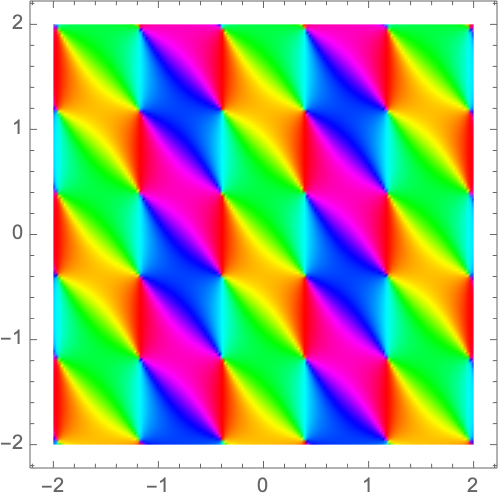Specify a collection of curves:

 In:=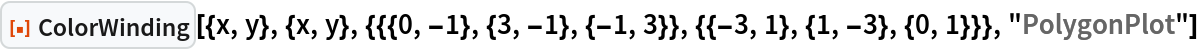Out=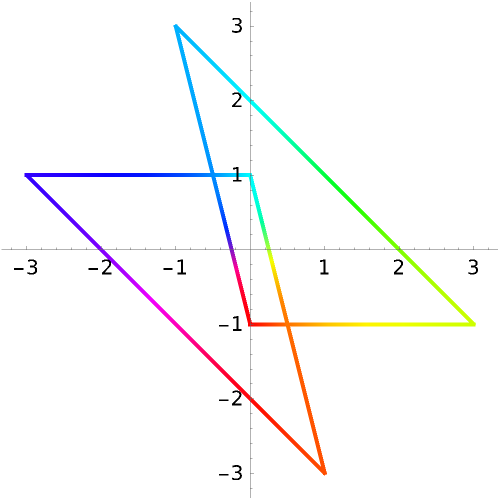All forms of Rectangle are recognized as valid lists of points:

 In:=Out=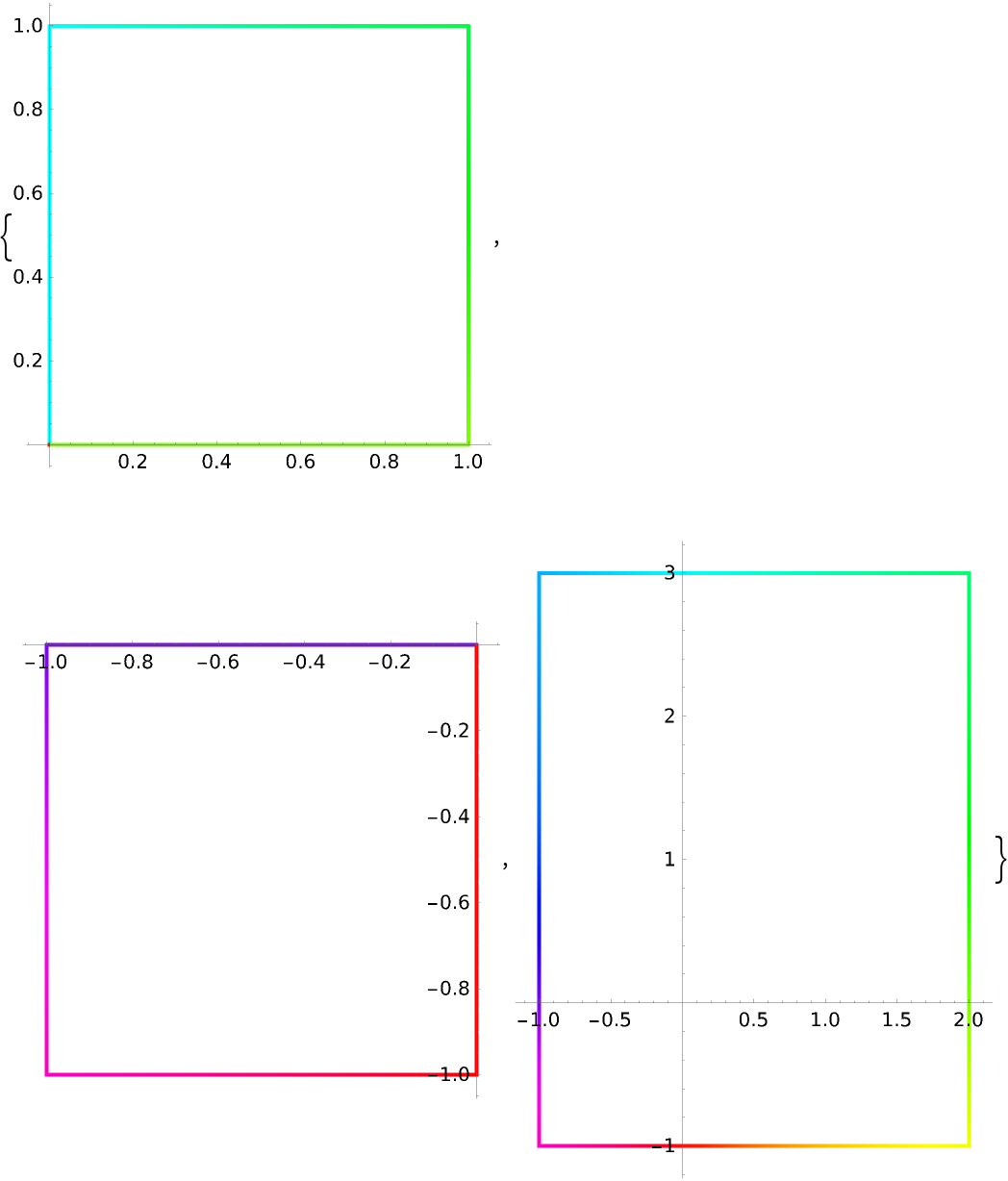All forms of Triangle are also supported:

 In:=Out=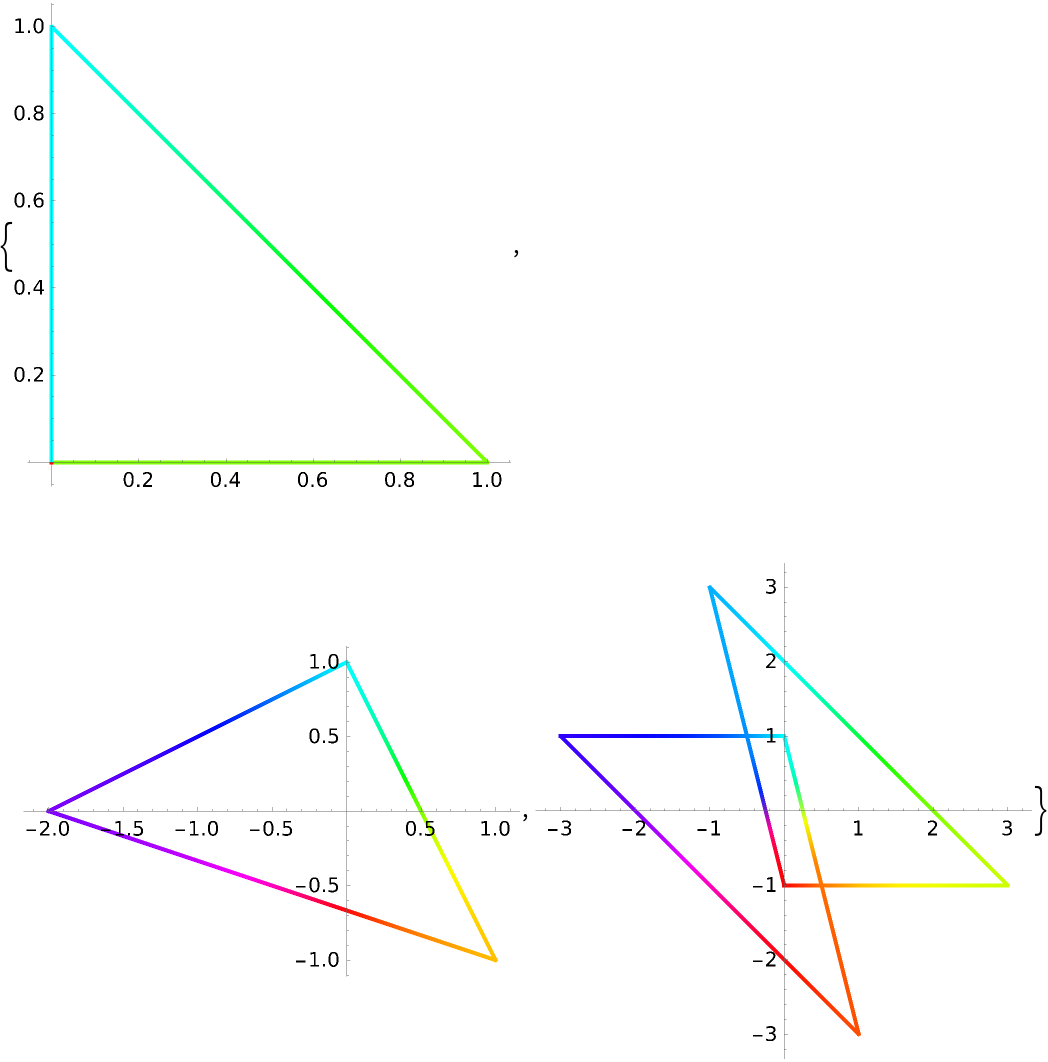The heads Polygon and Line are supported:

 In:=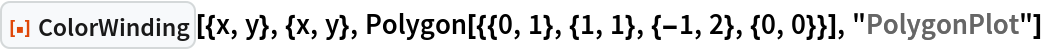Out=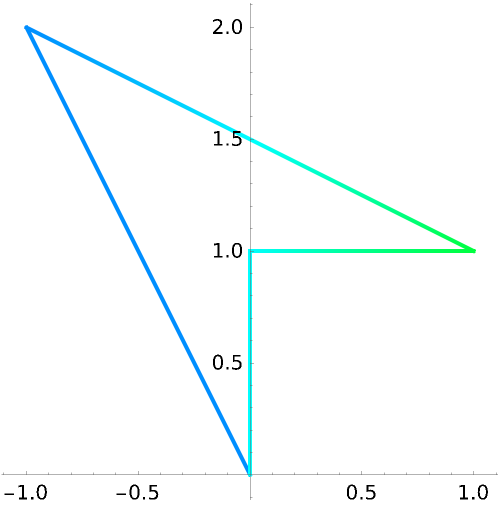In:=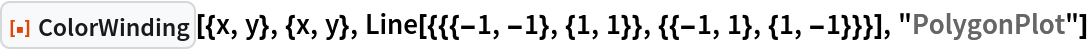Out=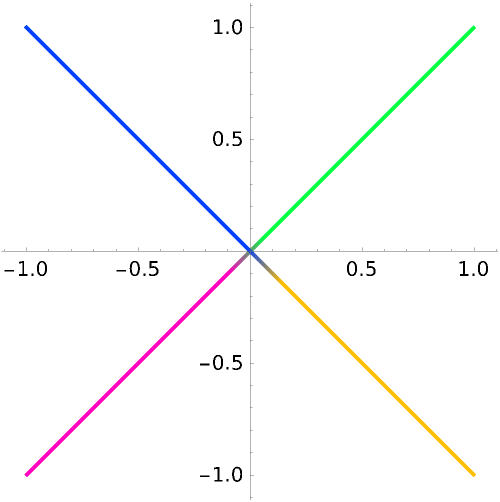Multiple output types can be selected in a list and their input order is reflected in the output:

 In:=Out=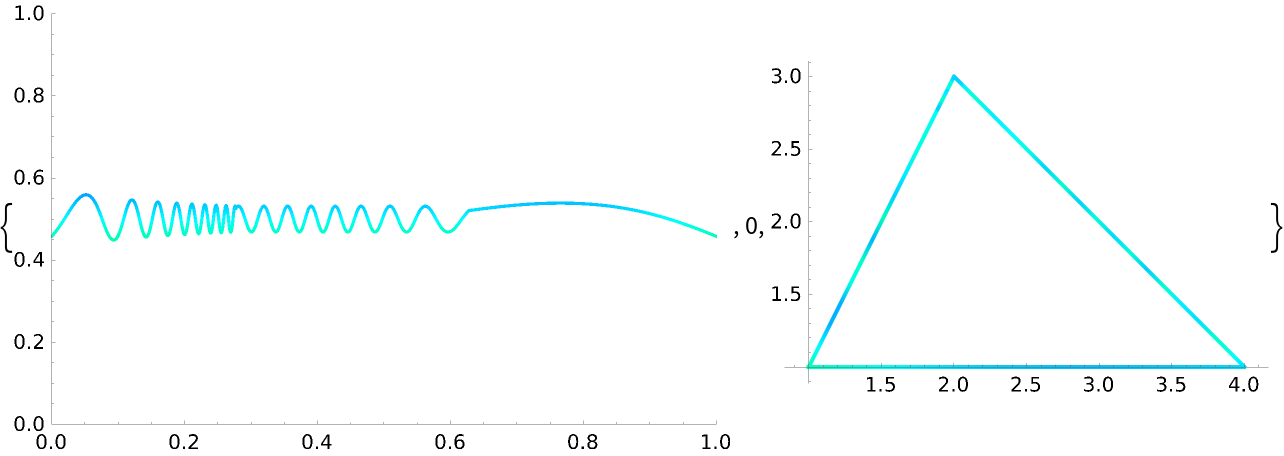If All is used as the output type, the function returns an Association:

 In:=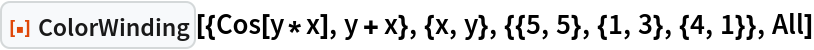Out=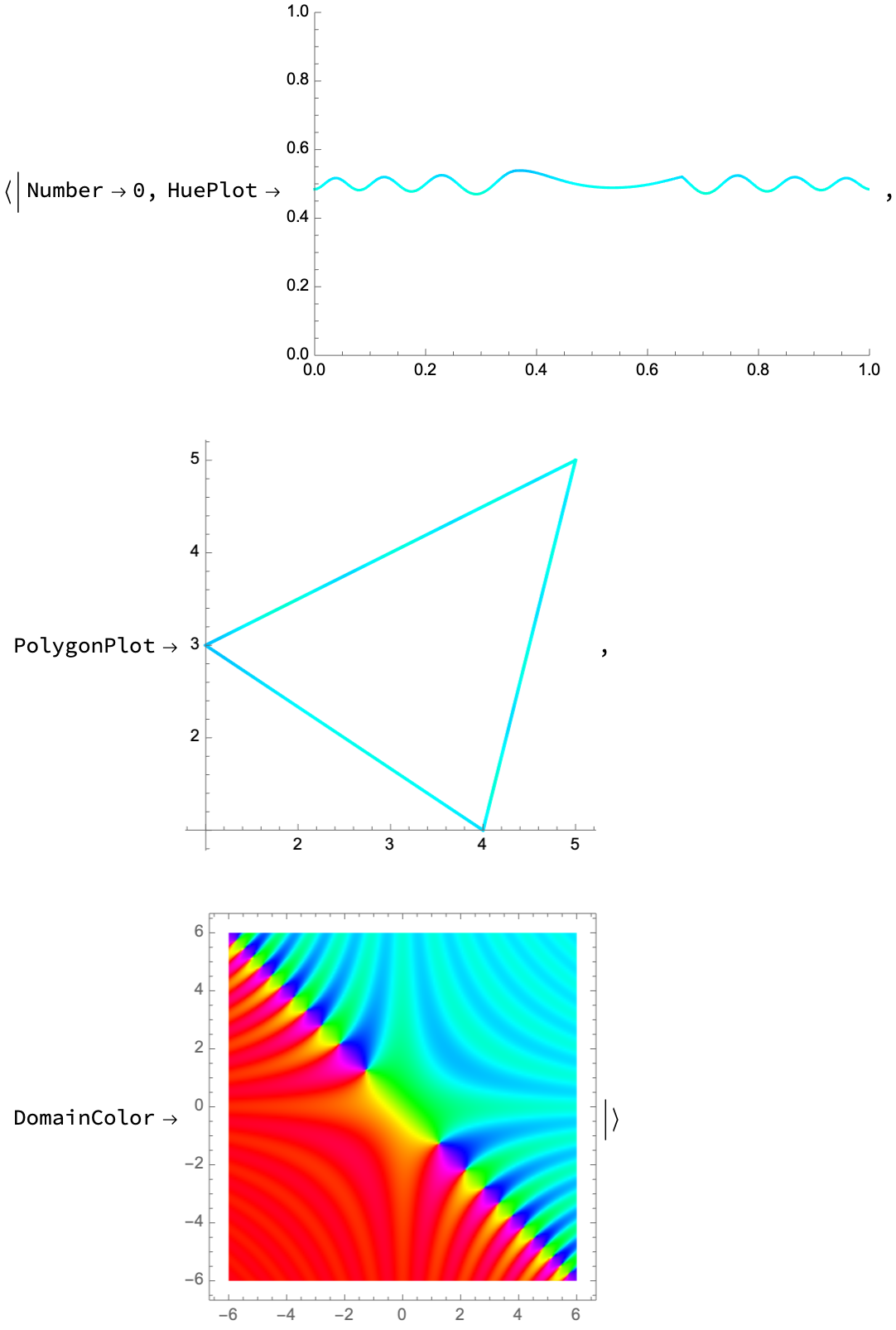### Options (5)

Determine if the list of points defines a closed curve:

 In:=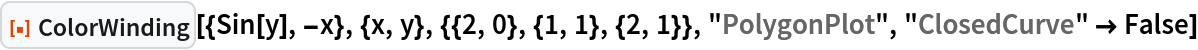Out=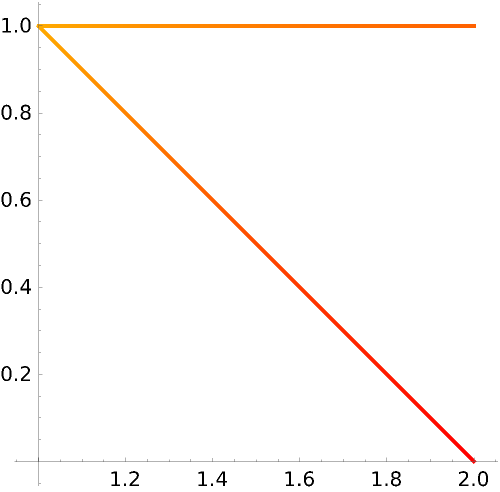The "IterationLength" option determines how frequently the Hue value is sampled along the curve, which produces more accurate results:

 In:=Out=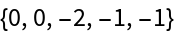The actual winding number is -1, as seen by inspecting the jumps in the below plot:

 In:=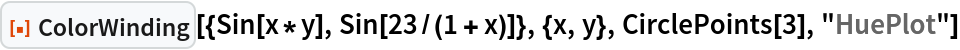Out=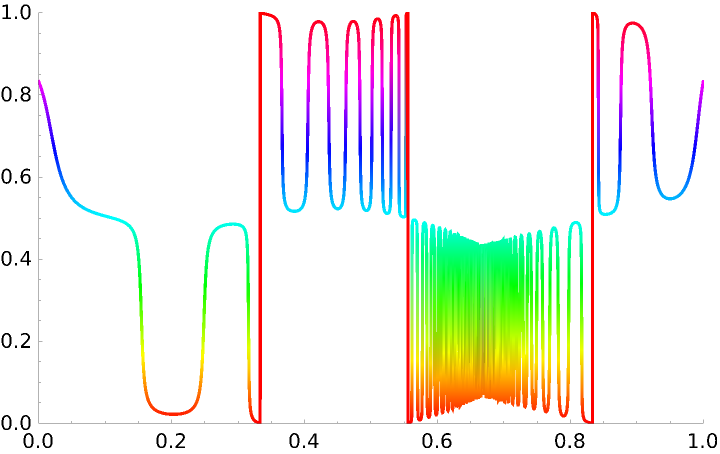The color wheel can be shifted by a selected amount:

 In:=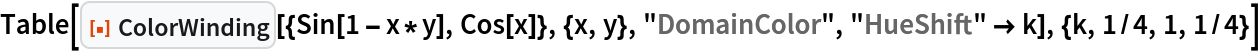Out=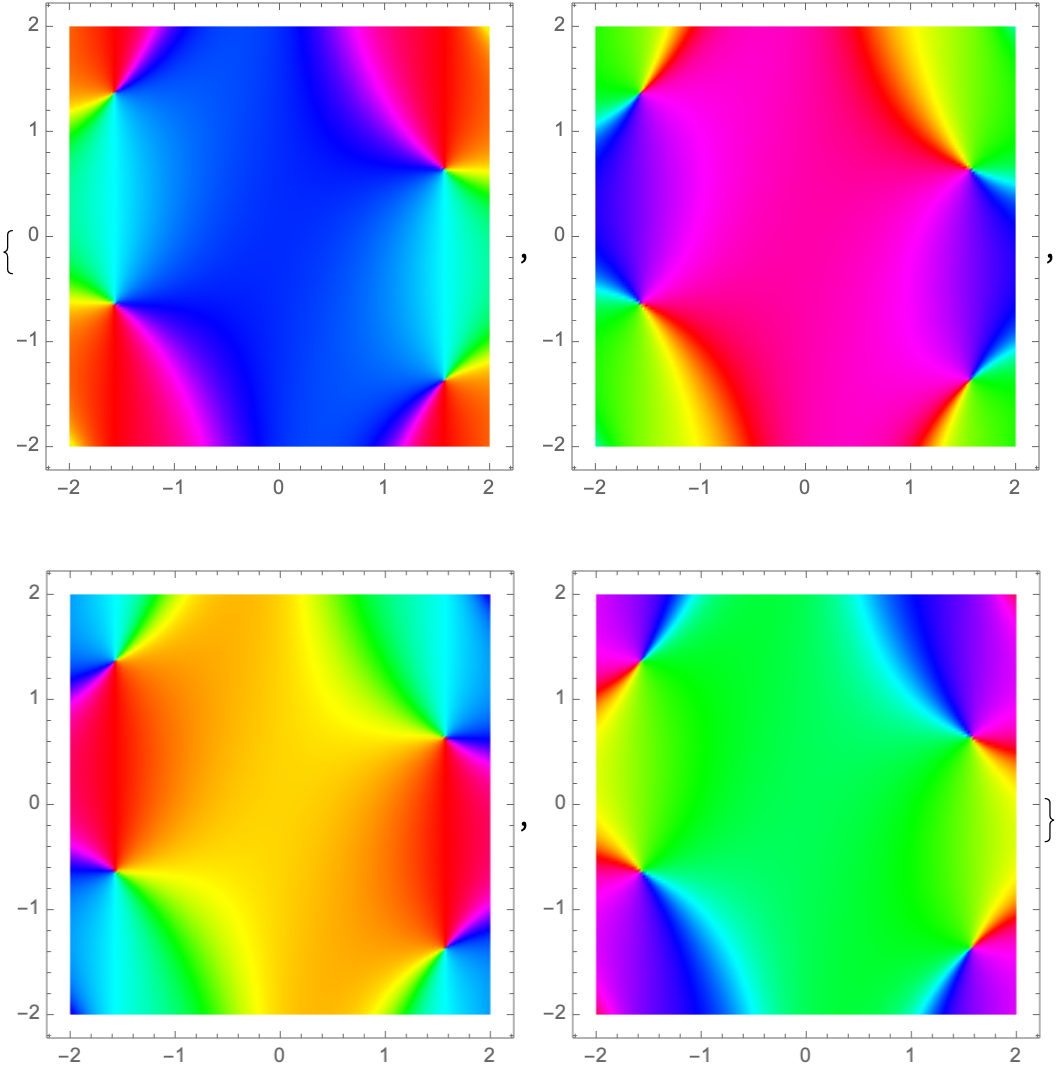Only the decimal value affects the hue shift:

 In:=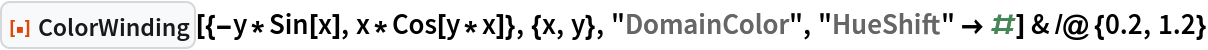Out=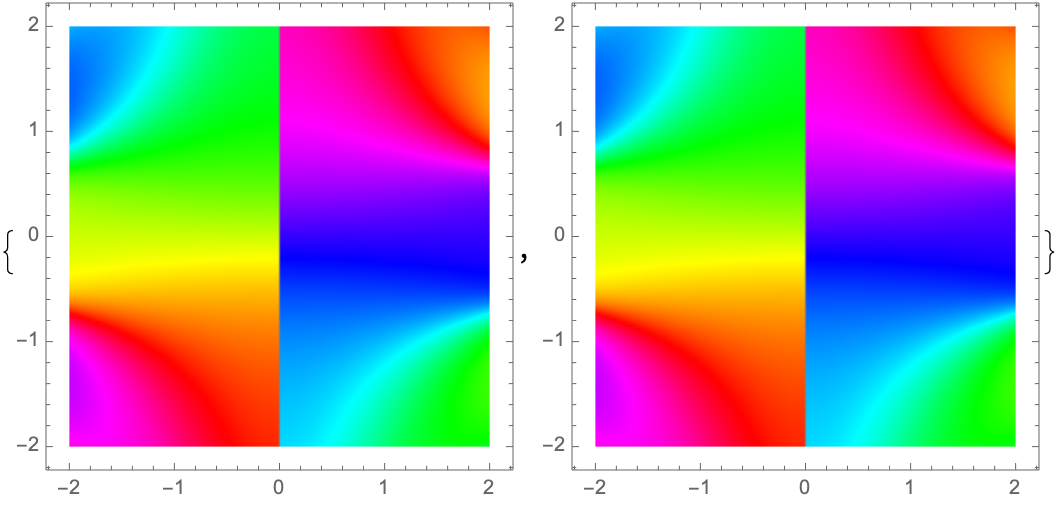The value of "IterationLength" determines how frequently the Hue value is sampled, as visualized below:

 In:=Out=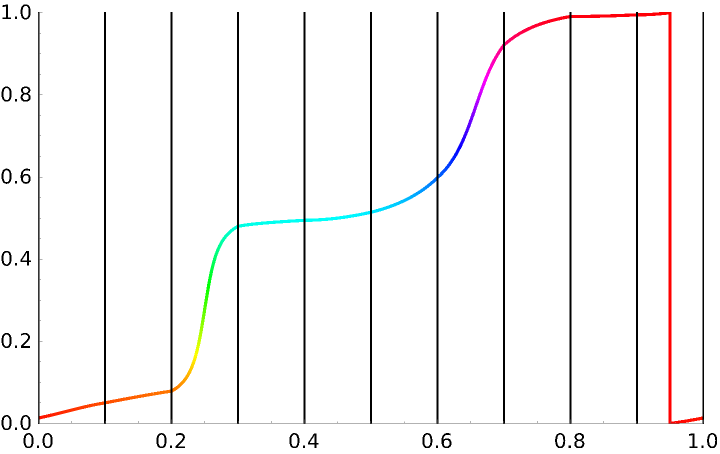The options for ParametricPlot are also supported:

 In:=Out=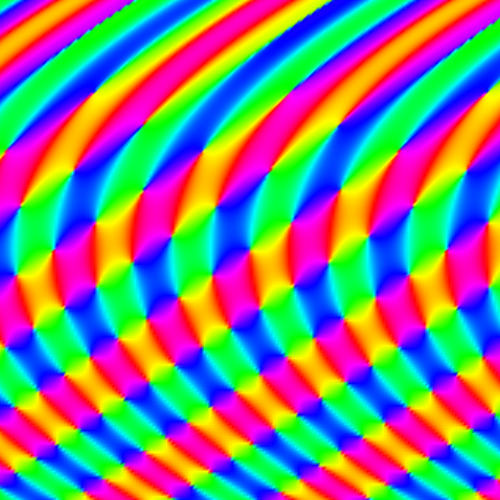### Applications (5)

For continuous transformations, any closed curve with a nonzero winding number contains a zero of the transformation:

 In:=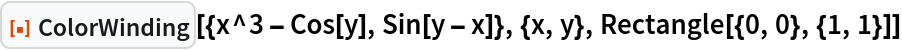Out=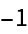If a boundary with nonzero winding number is halved, one half must also have a nonzero winding number:

 In:=Out=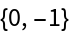Locate the zero by repeatedly decreasing the boundary size, ignoring areas where the winding number is zero:

 In:=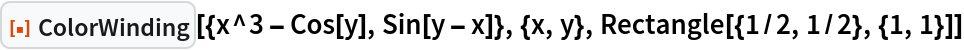Out=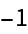In:=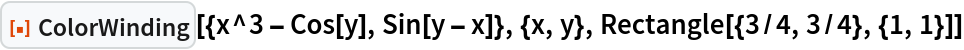Out=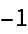In:=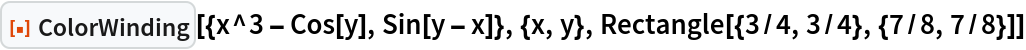Out=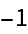The resulting boundary after repeating for a total of 10 times:

 In:=Out=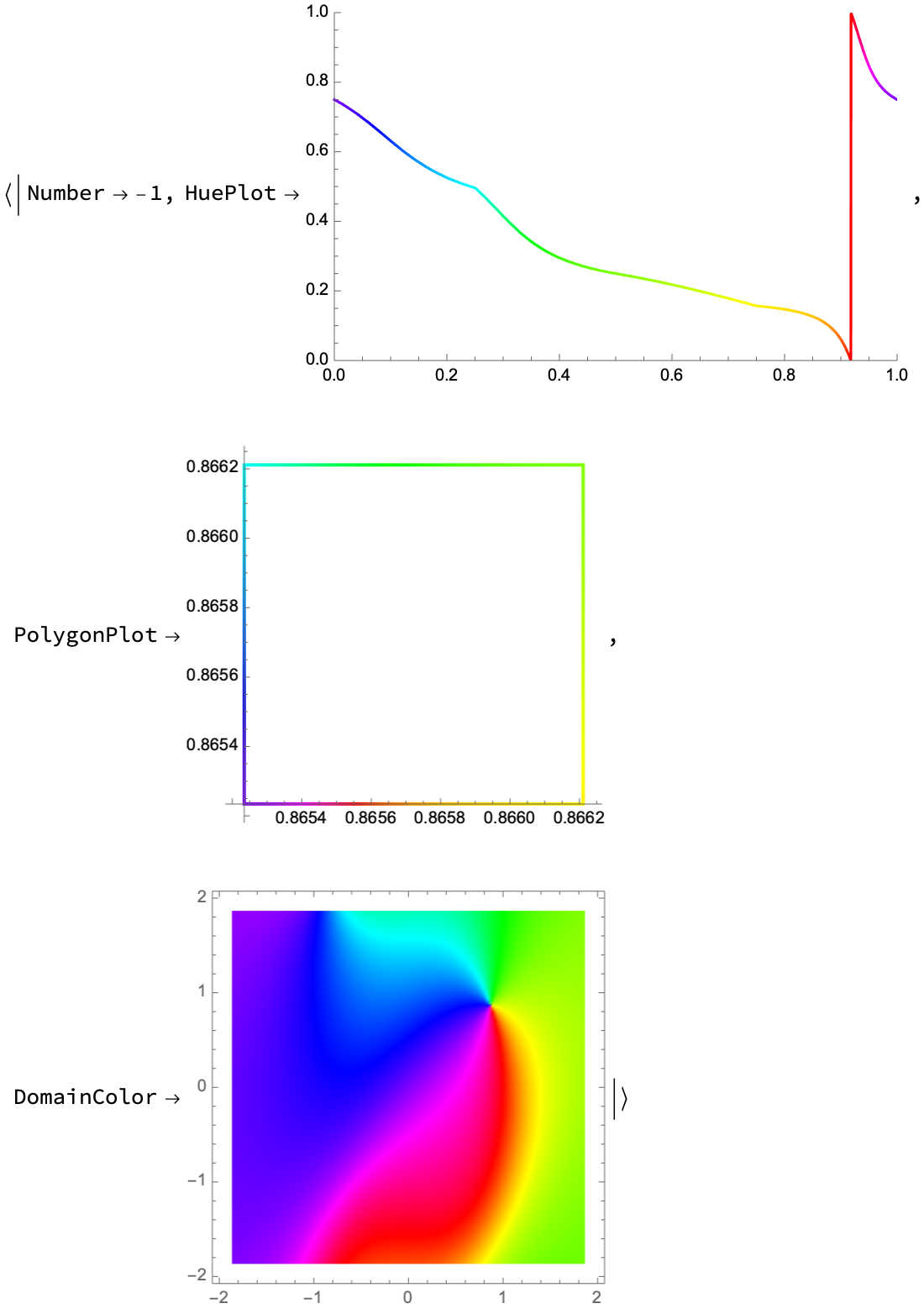The midpoints of the boundaries approach a zero of the transformation:

 In:=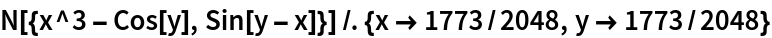Out=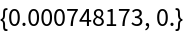### Properties and Relations (2)

Vector plots provide another visualization of 2D transformations:

 In:=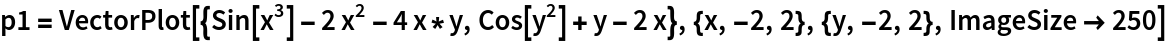Out=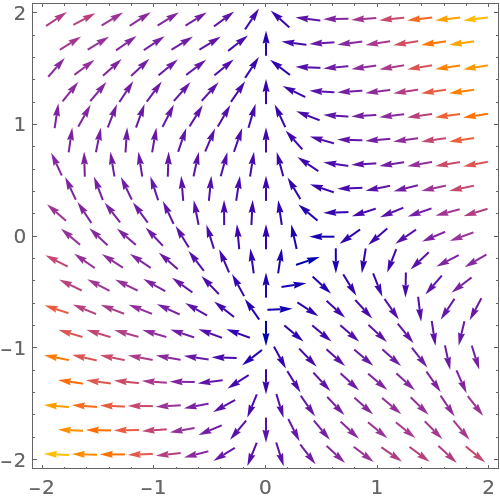Shown together, the similarities between vector plots and domain coloring become apparent:

 In:=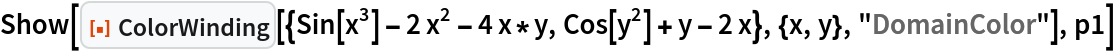Out=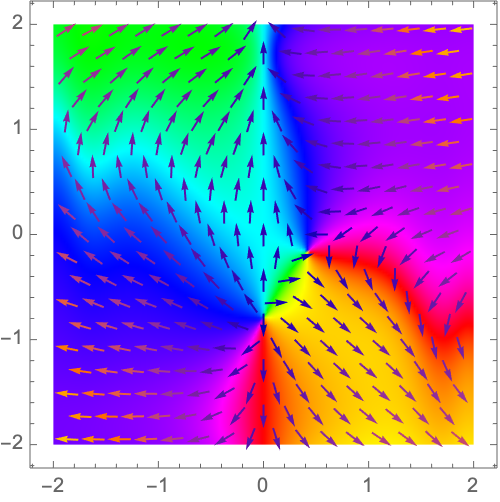### Possible Issues (3)

Discontinuities may occur in the color winding of a curve passing through a zero of the transformation:

 In:=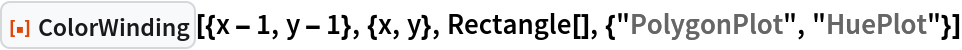Out=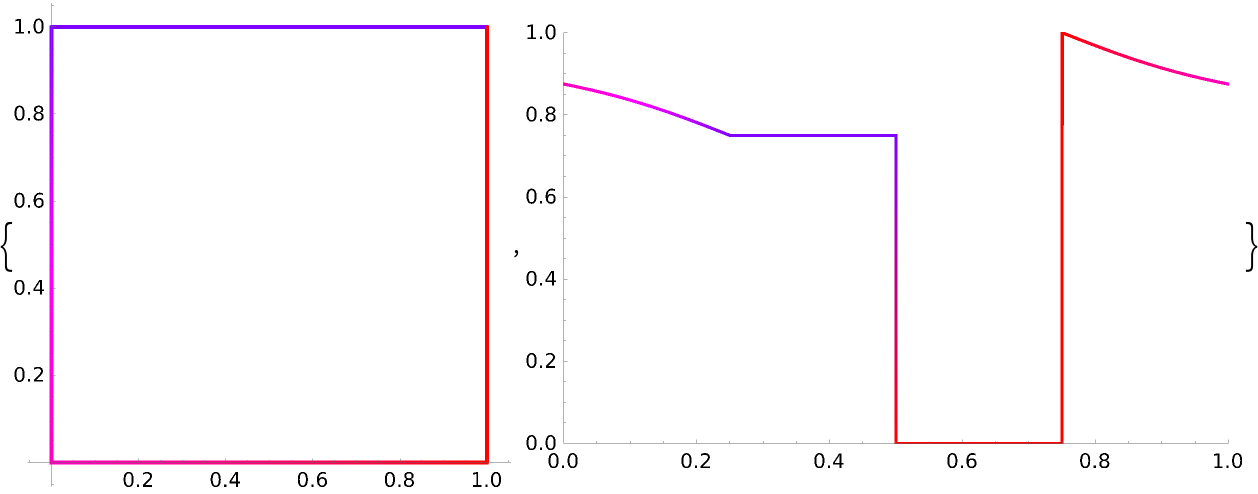A similar effect occurs for a curve passing though any asymptotes of the transformation:

 In:=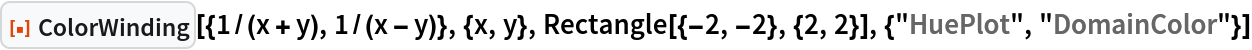Out=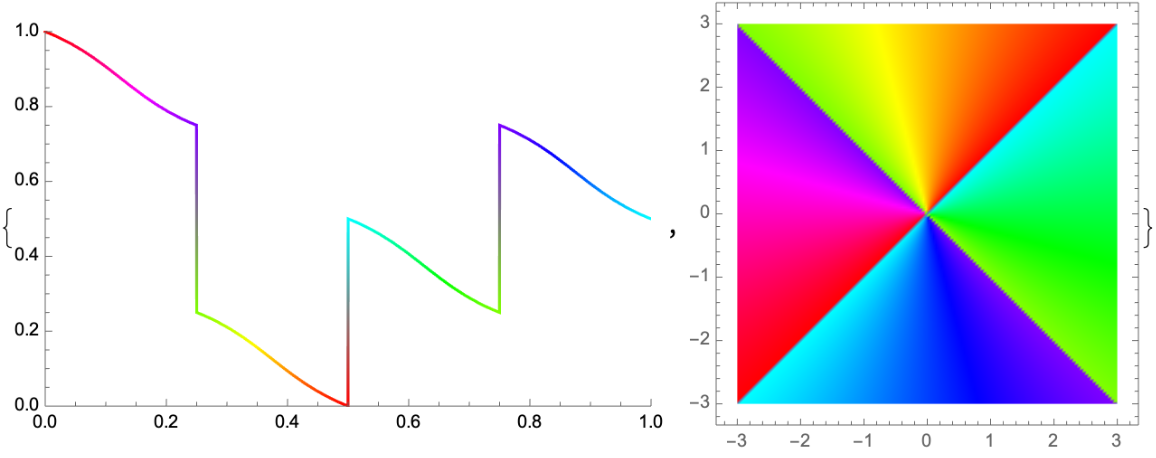Low values of the "IterationLength" option may produce inaccurate results:

 In:=Out=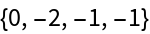Increasing the value of PlotPoints improves the resolution of the domain coloring:

 In:=Out=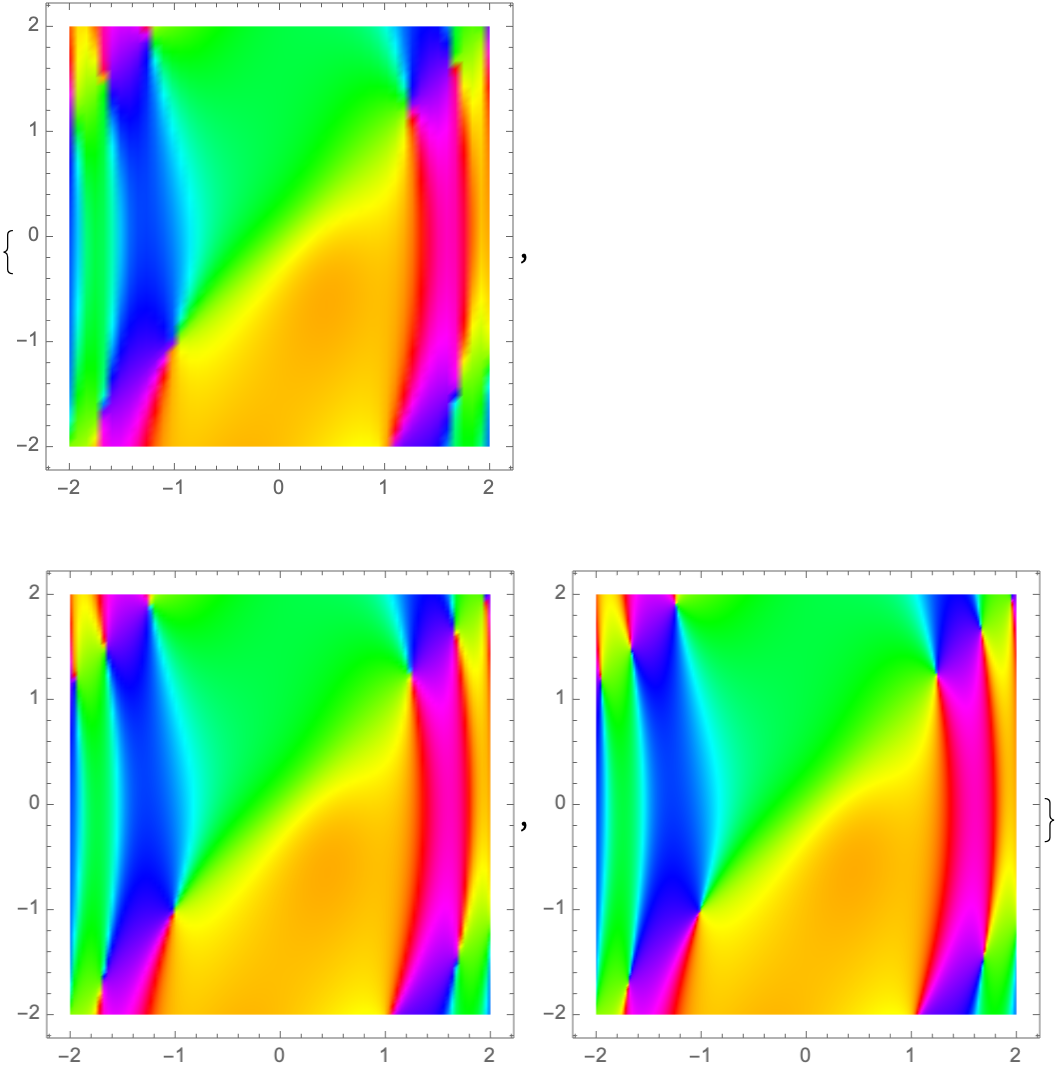### Neat Examples (4)

Create neat shapes by overlapping several domain coloring curves:

 In:=Out=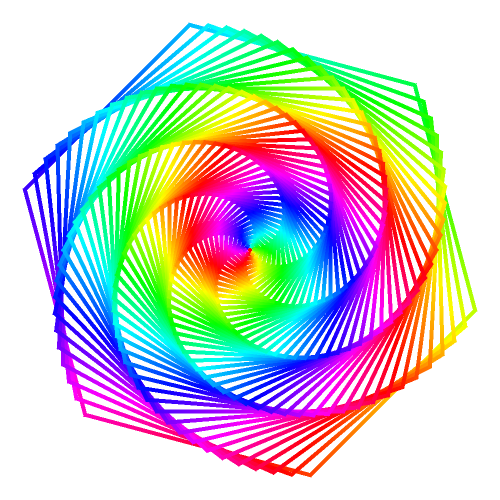Visualize how changing the transformation alters the domain coloring:

 In:=Out=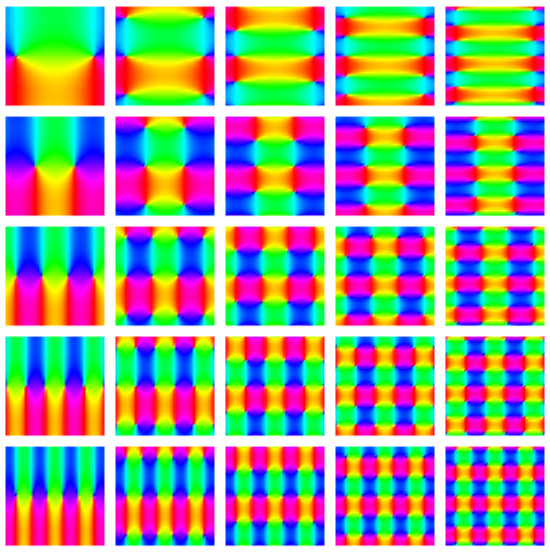Draw the domain coloring using a collection of triangular boundaries:

 In:=Out=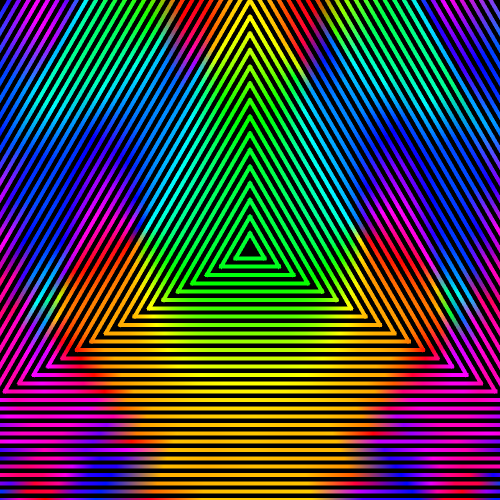Find the winding number along famous curves:

 In:=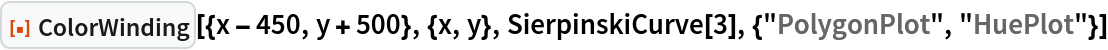Out=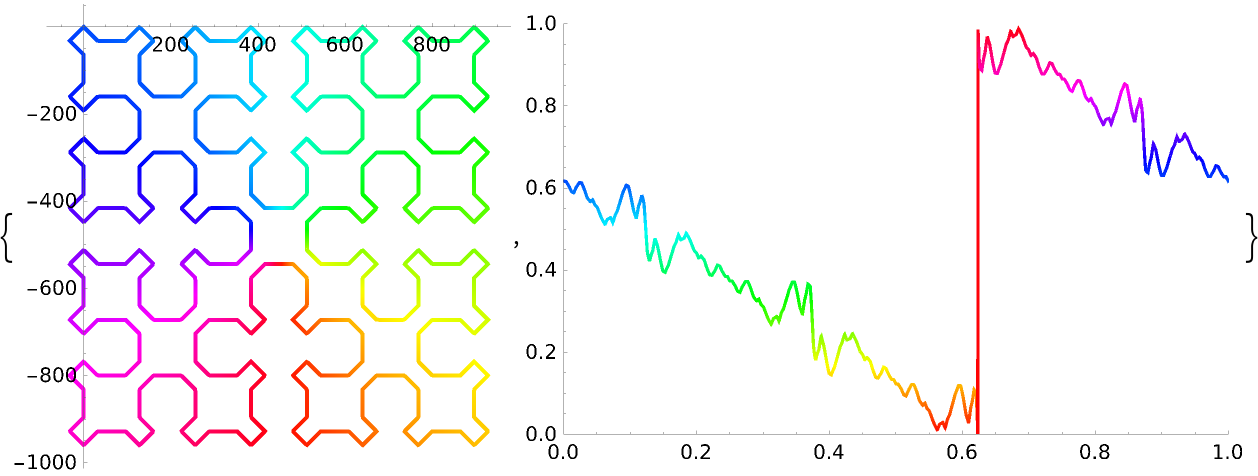In:=Out=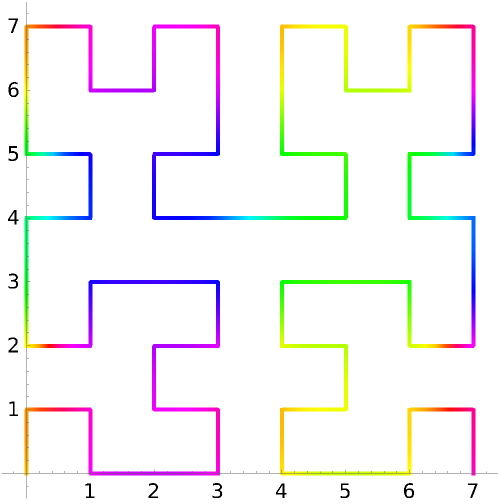## Publisher

Wolfram|Alpha Math Team

## Version History

• 2.0.0 – 23 March 2023
• 1.0.0 – 29 August 2022

## Author Notes

To view the full source code for ColorWinding, evaluate the following: